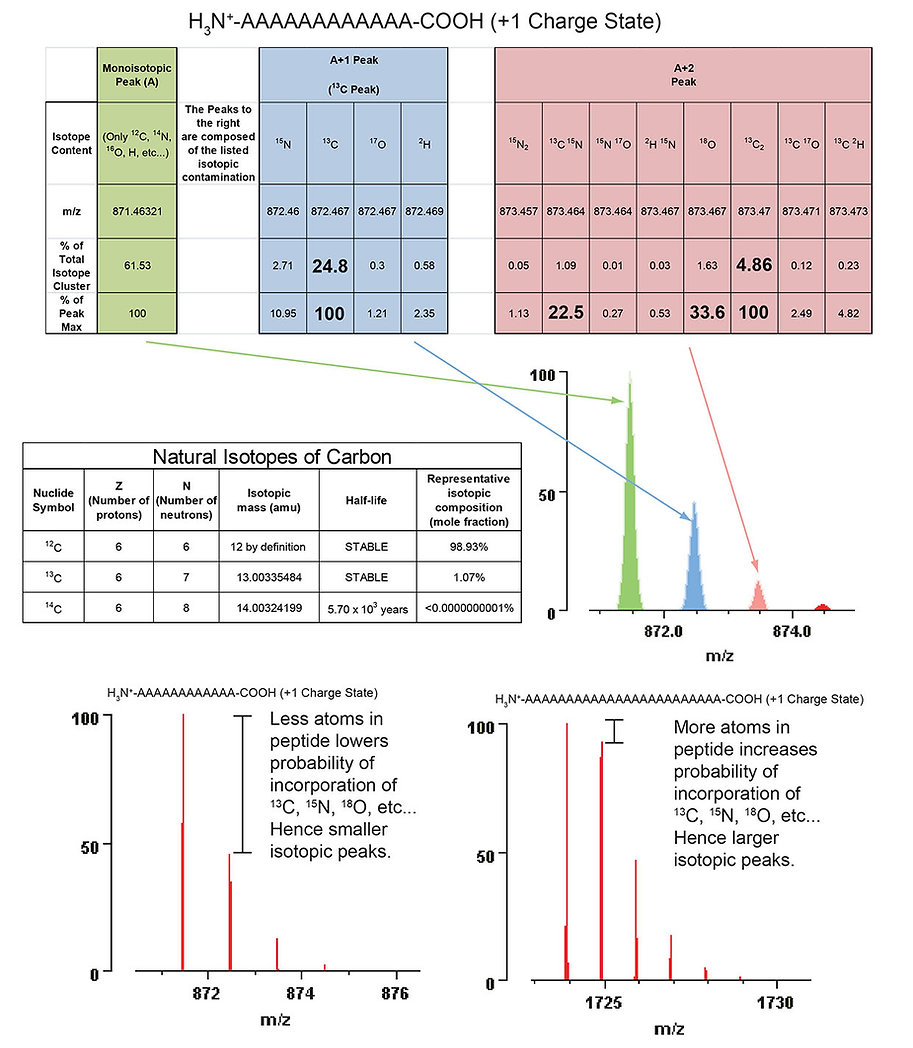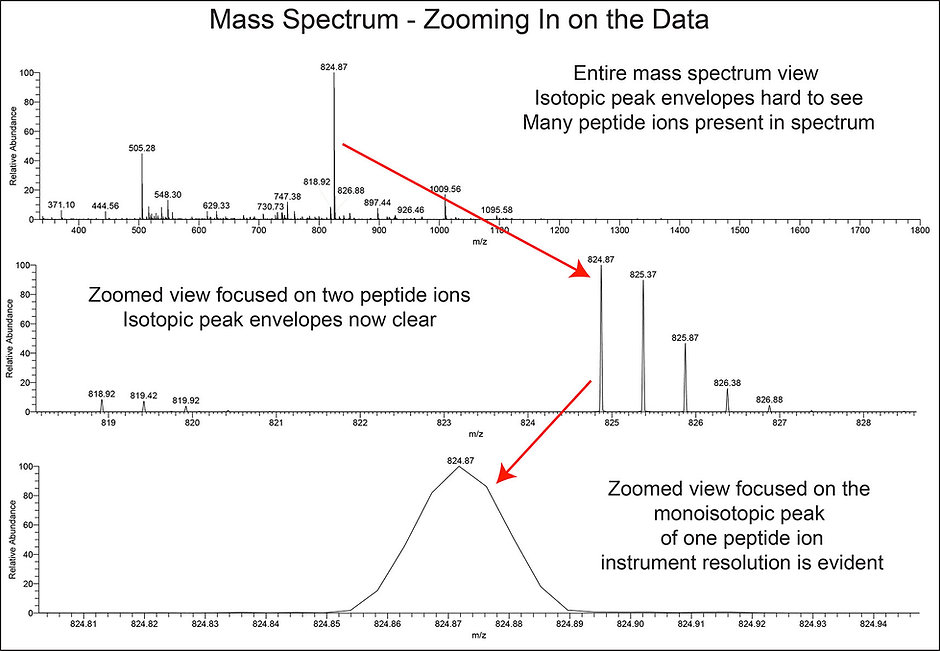## Isotopes and Isotopic Peaks

Isotopic peaks as shown in the spectra below can be seen for all molecules produced without artificial isotope enrichment. One isotope of carbon (carbon-13 or 13C) contributes significantly to the generation of these peaks. This occurs  because of the high natural abundance of 13C  on earth.# What is a mass spectrum?Every mass spectrum generated by a mass spectrometer can contain a large amount of data describing the intensity of ions detected versus their mass-to-charge ratio. This can been seen in the figure above, which shows a series of views with different zoom of the same mass spectrum obtained from a high resolution mass analyser.

To determine the full width at half-maximum (FWHM) resolution of a peak in a mass spectrum divide the m/z of the peak by the width of that peak at 50% of its intensity. An value of this number that is greater than 10,000 will give excellent separation of isotopic peaks. For the spectrum above the peak at m/z 824.87 has a FWHM resolution of 47,000.

## How can we calculate the charge state of a peptide ion?

A useful advantage of using high resolution mass analysers is the ability to determine the charge state of a peptide ion by calculating the m/z difference between its isotopic peaks. This information will allow us to calculate the monoisotopic mass (molecular weight) of the peptide being ionised. To make this calculation we use three pieces of information. First we know that the mass difference between the isotopic peaks is 1 amu  (1 Da) as isotopes differ in mass by the addition of 1 neutron which weighs 1 amu. Second if we have sufficient resolution we can determine the mass difference between two isotopic peaks the peptide ion. Usually the difference between the two most abundant isotopic peaks is chosen for accuracy. Third and finally we know that peptide ions become positively charged by the addition of protons which have a mass of 1 amu. The charge state of the peptide ion can be calculated for the isotopic envelope shown below. We can see that the m/z difference between the isotopic peaks is 0.25 and we know the mass difference between them is 1 amu therefore the charge state is +4. To calculate the peptide ion’s mass we multiply the m/z for the monoisotopic peak by the charge state. We then subtract the mass of the 4 protons which made the peptide ion positively charged.What can also be seen in the figure is that using a lower resolution mass analyser such as an iontrap compared here to a high resolution orbitrap makes charge state determination more difficult. This occurs because the isotopic peaks will blend into one another with low resoution mass analysers. Therefore in low resolution spectra the monoisotopic mass is not calculated but only the average mass is calculated (derived from the average of the isotopic peaks weighted by intensity).
The greatest advantage of knowing the charge state of every ion in a mass spectrum when trying to analyse peptides is that peptides due to their basic amino-terminus and prevelance of basic amino acids can stabilise more positive charge than other contaminating molecules such as polymers. When using electrospray ionisation this means that peptide ions are most abundant in the +2 and +3 charge states, while polymers and other contaminants are usually in the +1 charge state and can be ignored on this basis.

## What is a chromatogram?

When using online reverse phase liquid chromatography it is useful to display the data for an entire chromatographic separation over time in a graph known as a chromatogram. Shown below are three types of chromatogram used when analysing liquid chromatography mass spectrometry (LC-MS) data.
Using the mass spectrometers control software informations about the various peaks present may be extracted including integration of the peak area to give quantitative information, the retention time and the base peak for that chromatographic peak.